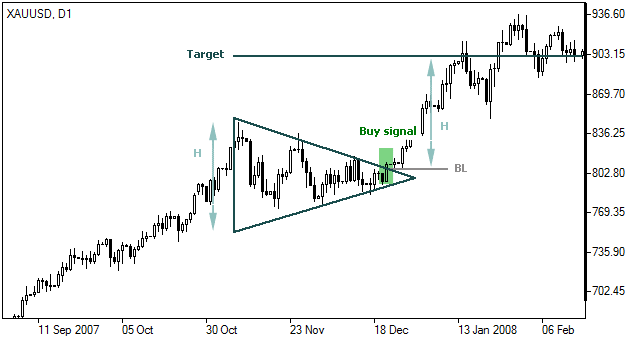# Symmetrical Triangle

The symmetrical triangle is a trading pattern that consists of simultaneous uptrend and downtrend lines. It shows a situation where supply and demand reached equilibrium or when a security’s price is consolidating.

Pattern Formation

This is identified as a price range of highs and lows narrowing to the extent that it forms a symmetric triangle positioned sideways. This type of pattern formation shows a descending resistance trendline and an ascending support trendline until the two meet at the center point. Both trendlines’ angles are almost of the same degree.

Photo taken from www.ifcmarkets.com

Interpretation of the Pattern

The Symmetric Triangle shows the movement and direction of the trend in case a breakout happens.

• When the triangle forms in a downtrend and the price breaks support, it is a good time to sell.
• When the triangle forms in an uptrend and the price breaks resistance, it is a good time to buy.

Symmetrical Triangle Formula

In Symmetrical Triangle, a target price is calculated based on the successive descending resistance trendline and ascending support trendline. In the pattern formation, the center point at which the two trendlines meet is considered as the target price. This target price is calculated as follows:

In case of an uptrend:

T = BL + H

In case of a downtrend:

T = BL – H

Where:

T – target price;

BL – breakthrough level (point where the price leaves the triangle);

H – pattern’s height (distance between support and resistance lines at pattern’s origin)